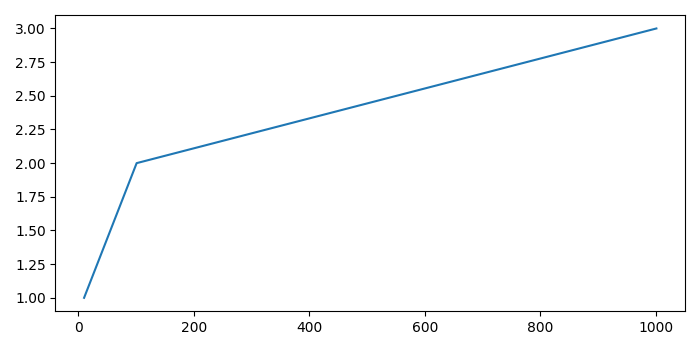# How to remove relative shift in Matplotlib axis?

To remove relative shift in matplotlib axis, we can take the following steps −

• Plot a line with two input lists.

• Using gca() method, get the current axis and then return the X-axis instance. Get the formatter of the major ticker. To remove the relative shift, use set_useOffset(False) method.

• To display the figure, use show() method.

## Example

from matplotlib import pyplot as plt
plt.rcParams["figure.figsize"] = [7.00, 3.50]
plt.rcParams["figure.autolayout"] = True
plt.plot([10, 101, 1001], [1, 2, 3])
plt.gca().get_xaxis().get_major_formatter().set_useOffset(False)
plt.show()

## Output